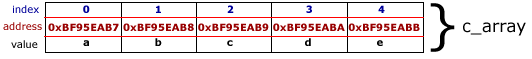In the previous post, “Pointers in C/C++”, we talked about pointers in C/C++. We recommend to read this posting before continuing.

Lets say we declare an array of 5 elements:

`int i_array;`

Also, we could declare and instantiate each elements in the array at the same time:

`int i_array = {10, 20, 30, 40, 50};`

Now, lets assume we created the array and we want to change the values of each element, one way could be:

```i_array = 15;
i_array = 25;
i_array = 35;
i_array = 45;
i_array = 55;```

However, there is another way to change the values of elements in an array, and that way is by using pointers.

To begin with, first we need to understand how are the array build, each element in the array have an address in memory, the name that we give to the array is connected to the address of the first element. Each consecutive elements will have an address that is the previous address plus the size of the address. For example, if you have an array of characters, each character have a size of 8 bits (1 byte), this means that each element’s address will be different by 1 byte.

For example, this  code would print the address of each element in an array:

```int main(int argc, char* argv[]){
int c_array = {'a', 'b', 'c', 'd', 'e'};
int index;
for (index = 0; index < 5; ++index){
printf('c_array[%d] with value %d has address 0x%X \n',
index, c_array[index], (unsigned int) &c_array[index]);
}
return 0;
}```

This will show us:

```c_array with value a has address 0xBF95EAB7
c_array with value b has address 0xBF95EAB8
c_array with value c has address 0xBF95EAB9
c_array with value d has address 0xBF95EABA
c_array with value e has address 0xBF95EABB```

As you can see they are separated by one byte:

`0xBF95EAB7 - 0xBF95EAB8 = 1`

The graphic representation of this array is:When working with pointers, we can access to the information indirectly by just using the address of each element in the array. The following code will make a pointer to point at the first element of the array:

`char* p_c_array = c_array; /* Not need &. c_array return an address. c_array return a value */`

The reason we are not using & when assigning the address is that arrays are accessed by reference, which means that the compiler will return the address of the first element if we write c_array while if we write c_array it will return the value in that position.

If we want to print the first element:

`printf('First Element: %c', *p_c_array);`

If we want to print the second element, we need to increase the pointer so it will point to the next element.
By increasing it means that we must increase a total amount of one byte (because we are talking about char variables) to access to the next element:

`0xBF95EAB7 + 1 byte =  0xBF95EAB8 `

There are two ways to increase value in the pointer by one byte:
One ways is:

`p_c_array = p_c_array + sizeof(char); /* Size of char return 1 byte */`

Or by letting the compiler to increase the value:

`p_c_array++;`

Just take in consideration that you don’t wish to loose the original address to which you are pointing to the first element of the array; therefore it common practice to create a second pointer that will have the same address and increase that pointer, for example:

```char* p_c_array = c_array;  /* Point to first element of array */
char* p_c_array_2;```
```p_c_array2 = p_c_array;      /* Copy address stored in p_c_array */
p_c_array2++;                /* Increase address stored by one byte to point to next element */
printf('First element: %c. Second Element %c \n',
*p_c_array,
*p_c_array2);```

The follow example would print all the elements of the array:

```int main(int argc, char* argv[]){
char c_array = {'a', 'b', 'c', 'd', 'e'};
char* p_c_array = c_array;
char* p_c_array_2;
for (p_c_array_2 = p_c_array;
*p_c_array_2 != '\0';
++p_c_array_2){
printf('p_c_array_2 points to variable with value %c \n',
*p_c_array_2);
}
return 0;
}```

Notice that we are using in this case ‘\0’ to indicate the end of the array. This do not apply if we would be using an array of integers for example.

This code will print to screen:

```p_c_array_2 points to variable with value a
p_c_array_2 points to variable with value b
p_c_array_2 points to variable with value c
p_c_array_2 points to variable with value d
p_c_array_2 points to variable with value e```